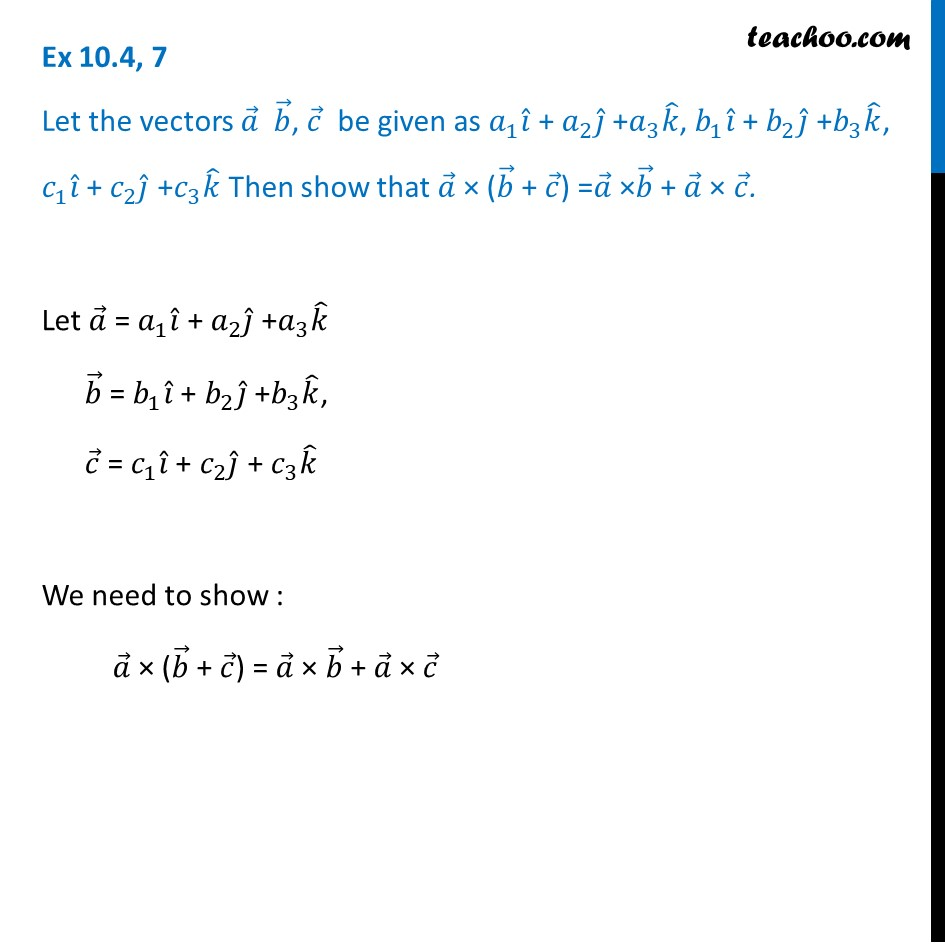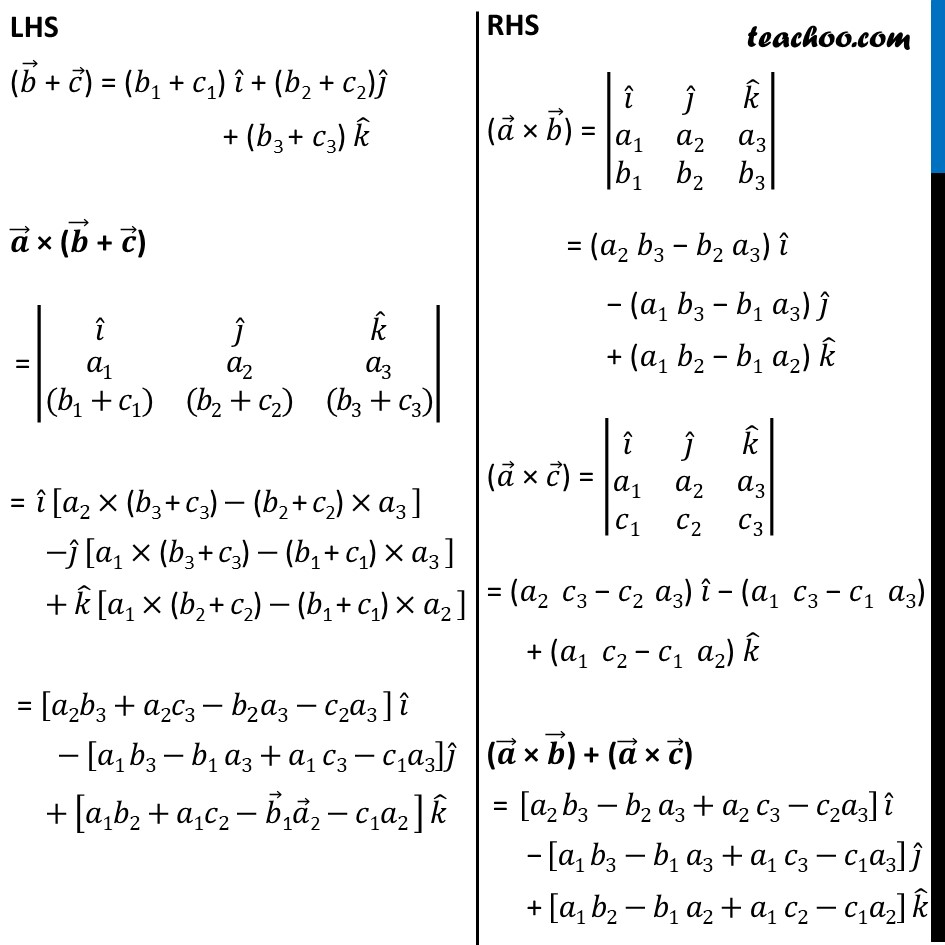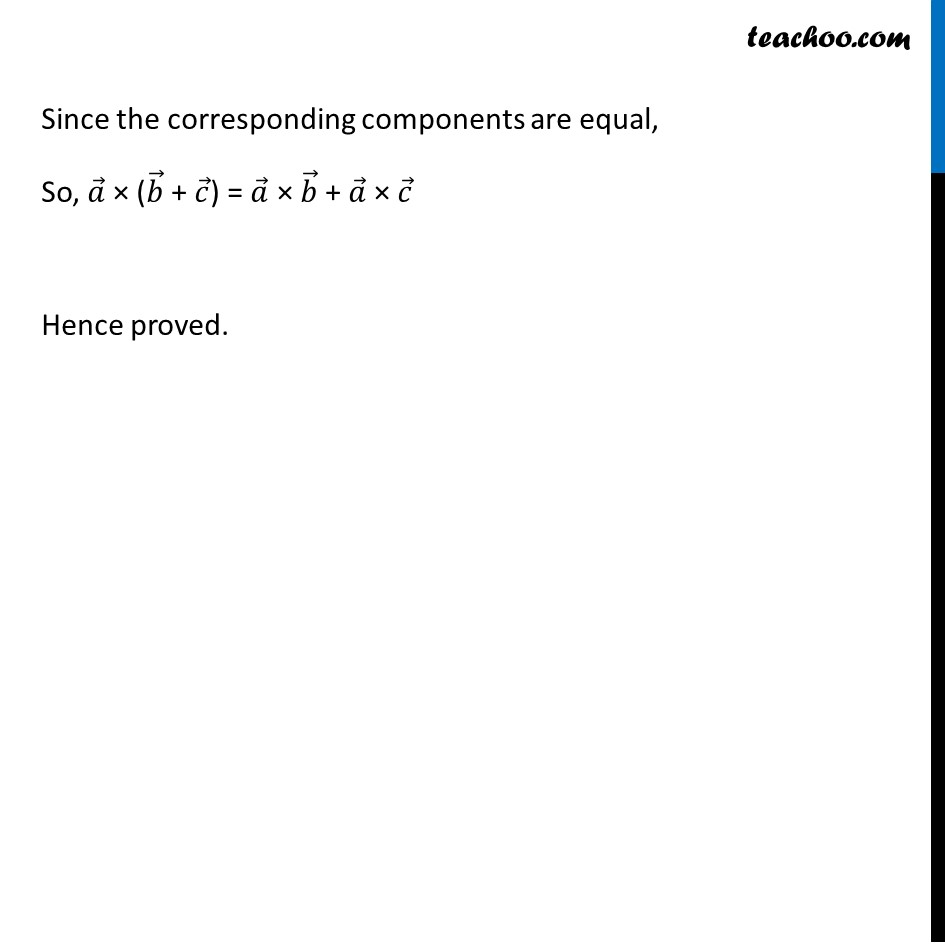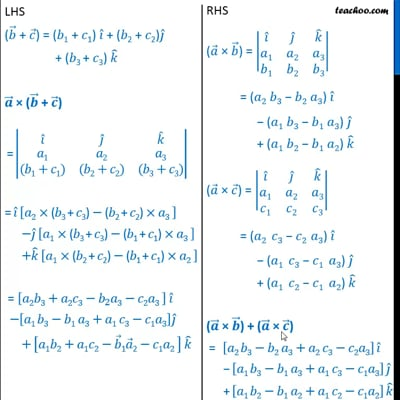Ex 10.4

Chapter 10 Class 12 Vector Algebra
Serial order wiseThis video is only available for Teachoo black users

Introducing your new favourite teacher - Teachoo Black, at only ₹83 per month

### Transcript

Ex 10.4, 7 Let the vectors 𝑎 ⃗ 𝑏 ⃗, 𝑐 ⃗ be given as 𝑎_1 𝑖 ̂ + 𝑎_2 𝑗 ̂ +𝑎_3 𝑘 ̂, 𝑏_1 𝑖 ̂ + 𝑏_2 𝑗 ̂ +𝑏_3 𝑘 ̂, 𝑐_1 𝑖 ̂ + 𝑐_2 𝑗 ̂ +𝑐_3 𝑘 ̂ Then show that 𝑎 ⃗ × (𝑏 ⃗ + 𝑐 ⃗) =𝑎 ⃗ ×𝑏 ⃗ + 𝑎 ⃗ × 𝑐 ⃗. Let 𝑎 ⃗ = 𝑎_1 𝑖 ̂ + 𝑎_2 𝑗 ̂ +𝑎_3 𝑘 ̂ 𝑏 ⃗ = 𝑏_1 𝑖 ̂ + 𝑏_2 𝑗 ̂ +𝑏_3 𝑘 ̂, 𝑐 ⃗ = 𝑐_1 𝑖 ̂ + 𝑐_2 𝑗 ̂ + 𝑐_3 𝑘 ̂ We need to show : 𝑎 ⃗ × (𝑏 ⃗ + 𝑐 ⃗) = 𝑎 ⃗ × 𝑏 ⃗ + 𝑎 ⃗ × 𝑐 ⃗ RHS (𝑎 ⃗ × 𝑏 ⃗) = |■8(𝑖 ̂&𝑗 ̂&𝑘 ̂@𝑎1&𝑎2&𝑎3@𝑏1&𝑏2&𝑏3)| = (𝑎2 𝑏3 − 𝑏2 𝑎3) 𝑖 ̂ − (𝑎1 𝑏3 − 𝑏1 𝑎3) 𝑗 ̂ + (𝑎1 𝑏2 − 𝑏1 𝑎2) 𝑘 ̂ (𝑎 ⃗ × 𝑐 ⃗) = |■8(𝑖 ̂&𝑗 ̂&𝑘 ̂@𝑎1&𝑎2&𝑎3@𝑐1&𝑐2&𝑐3)| = (𝑎2" " 𝑐3 − 𝑐2" " 𝑎3) 𝑖 ̂ − (𝑎1" " 𝑐3 − 𝑐1" " 𝑎3) 𝑗 ̂ + (𝑎1" " 𝑐2 − 𝑐1" " 𝑎2) 𝑘 ̂ (𝒂 ⃗ × 𝒃 ⃗) + (𝒂 ⃗ × 𝒄 ⃗) = [𝑎2" " 𝑏3−𝑏2 𝑎3+𝑎2 𝑐3−𝑐2𝑎3] 𝑖 ̂ − [𝑎1" " 𝑏3−𝑏1 𝑎3+𝑎1 𝑐3−𝑐1𝑎3] 𝑗 ̂ + [𝑎1" " 𝑏2−𝑏1 𝑎2+𝑎1 𝑐2−𝑐1𝑎2] 𝑘 ̂ Since the corresponding components are equal, So, 𝑎 ⃗ × (𝑏 ⃗ + 𝑐 ⃗) = 𝑎 ⃗ × 𝑏 ⃗ + 𝑎 ⃗ × 𝑐 ⃗ Hence proved. Ex 10.4, 7 Let the vectors , be given as 1 + 2 + 3 , 1 + 2 + 3 , 1 + 2 + 3 Then show that ( + ) = + . Let = 1 + 2 + 3 = 1 + 2 + 3 , = 1 + 2 + 3 We need to show : ( + ) = + LHS ( + ) = ( 1 + 1) + ( 2 + 2) + ( 3 + 3) ( + ) = 1 ( 1+ 1) 2 ( 2+ 2) 3 ( 3+ 3) = 2 ( 3 + 3) ( 2 + 2) 3 1 ( 3 + 3) ( 1 + 1) 3 + 1 ( 2 + 2) ( 1 + 1) 2 = 2 3+ 2 3 2 3 2 3 1 3 1 3+ 1 3 1 3 + 1 2+ 1 2 1 2 1 2